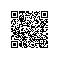# 从零开始定义自己的JavaScript框架(一)

## 1.1 模块的定义

JavaScript这种语言比较奇怪，奇怪在哪里呢，它的现有版本里没package跟class，要是有，我们也没必要来考虑什么自己做模块化了。那它是要用什么东西来隔绝作用域呢？

for(var i=0; i<5; i++){//do something}
alert(i);

(function(){
window.thin ={
define:function(name, dependencies, factory){//register a module}};})();

(function(){var moduleMap ={};
window.thin ={
define:function(name, dependencies, factory){if(!moduleMap[name]){varmodule={
name: name,
dependencies: dependencies,
factory: factory
};
moduleMap[name]=module;}return moduleMap[name];}};})();

## 1.2 模块的使用

thin.define("A",[],function(){//module A});

thin.define("B",["A"],function(A){//module Bvar a =new A();});

use:function(name){varmodule= moduleMap[name];if(!module.entity){var args =[];for(var i=0; i<module.dependencies.length; i++){if(moduleMap[module.dependencies[i]].entity){
args.push(moduleMap[module.dependencies[i]].entity);}else{
args.push(this.use(module.dependencies[i]));}}module.entity =module.factory.apply(noop, args);}returnmodule.entity;}

thin.define("D",["A","B","C"],function(A, B, C){//module Dvar a =new A();var b =new B();var c =new C();});

thin.define("foo.D",["foo.A","foo.B","foo.C"],function(A, B, C){//module Dvar a =new A();var b =new B();var c =new C();});

thin.define("A",[],function(){return"a";});

thin.define("B",[],function(){return"b";});

thin.define("C",[],function(){return"c";});

thin.define("D",["A","B","C"],function(B, A, C)   {return B + A + C;});var D = thin.use("D");
alert(D);

AngularJS使用了一种比较极端的办法，分析了函数的字面量。众所周知，在JavaScript中，任何对象都隐含了toString方法，对于一个函数来说，它的toString就是自己的实现代码，包含函数签名和注释。下面我贴一下AngularJS里面的这部分代码：

var FN_ARGS =/^function\s*[^$$]*\(\s*([^$$]*)\)/m;var FN_ARG_SPLIT =/,/;var FN_ARG =/^\s*(_?)(\S+?)\1\s*$/;var STRIP_COMMENTS =/((\/\/.*$)|(\/\*[\s\S]*?\*\/))/mg;function annotate(fn){var $inject, fnText, argDecl,last;if(typeof fn =='function'){if(!($inject = fn.$inject)){$inject =[];
argDecl = fnText.match(FN_ARGS);
forEach(argDecl.split(FN_ARG_SPLIT),function(arg){
arg.replace(FN_ARG,function(all, underscore, name){
$inject.push(name);});}); fn.$inject = $inject;}}elseif(isArray(fn)){last= fn.length -1; assertArgFn(fn[last],'fn');$inject = fn.slice(0,last);}else{
assertArgFn(fn,'fn',true);}return \$inject;}

AngularJS的这段代码很强大，但是要损耗一些性能，考虑到我们的框架首要原则是简单，甚至可以为此牺牲一些灵活性，我们不做这么复杂的事情了。

## 1.3 模块的加载

[补一段，@Franky 大神指出了这篇文章中一些不符合现状的地方，我把它也贴在这里，供读者参考]

require:function(pathArr, callback){for(var i =0; i < pathArr.length; i++){var path = pathArr[i];if(!fileMap[path]){var head = document.getElementsByTagName('head');var node = document.createElement('script');
node.type ='text/javascript';
node.async ='true';
node.src = path +'.js';
fileMap[path]=true;
checkAllFiles();};
callback();}}}

## 1.4 小结

(function(){var moduleMap ={};var fileMap ={};var noop =function(){};var thin ={
define:function(name, dependencies, factory){if(!moduleMap[name]){varmodule={
name: name,
dependencies: dependencies,
factory: factory
};

moduleMap[name]=module;}return moduleMap[name];},use:function(name){varmodule= moduleMap[name];if(!module.entity){var args =[];for(var i=0; i<module.dependencies.length; i++){if(moduleMap[module.dependencies[i]].entity){
args.push(moduleMap[module.dependencies[i]].entity);}else{
args.push(this.use(module.dependencies[i]));}}module.entity =module.factory.apply(noop, args);}returnmodule.entity;},require:function(pathArr, callback){for(var i =0; i < pathArr.length; i++){var path = pathArr[i];if(!fileMap[path]){var head = document.getElementsByTagName('head');var node = document.createElement('script');
node.type ='text/javascript';
node.async ='true';
node.src = path +'.js';
fileMap[path]=true;
checkAllFiles();};
callback();}}}};

window.thin = thin;})();

thin.define("constant.PI",[],function(){return3.14159;});

thin.define("shape.Circle",["constant.PI"],function(pi){varCircle=function(r){this.r = r;};Circle.prototype ={
area :function(){return pi *this.r *this.r;}}returnCircle;});

thin.define("shape.Rectangle",[],function(){varRectangle=function(l, w){this.l = l;this.w = w;};Rectangle.prototype ={
area:function(){returnthis.l *this.w;}};returnRectangle;});

thin.define("ShapeTypes",["shape.Circle","shape.Rectangle"],function(Circle,Rectangle){return{
CIRCLE:Circle,
RECTANGLE:Rectangle};});

thin.define("ShapeFactory",["ShapeTypes"],function(ShapeTypes){return{
getShape:function(type){var shape;switch(type){case"CIRCLE":{
shape =newShapeTypes[type](arguments);break;}case"RECTANGLE":{
shape =newShapeTypes[type](arguments, arguments);break;}}return shape;}};});varShapeFactory= thin.use("ShapeFactory");
alert(ShapeFactory.getShape("RECTANGLE",3,4).area());使用钉钉扫一扫加入圈子
+ 订阅